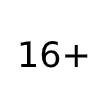DOI: 10.18413/2518-1092-2019-4-3-0-2

# ON QUASI-SUBBAND MATRICES OF COSINE TRANSFORM

In the article we explore the properties of quasi-subband cosine transform matrices used in subband analysis and synthesis of signals and images. It is shown that their eigenvalues can have positive and negative values; the estimates of their quantity are proposed. It is shown that the sum of quasi-subband matrices corresponding to the partition of the domain of definition of the cosine transform is equal to the zero matrix. In the article we demonstrate the examples of images subband components extracted by using quasi-subband matrices; the examples of the basic images obtained by using the product of the eigenvectors of the analysed matrices under study are given.

Number of views: 1206
Количество скачиваний: 5297